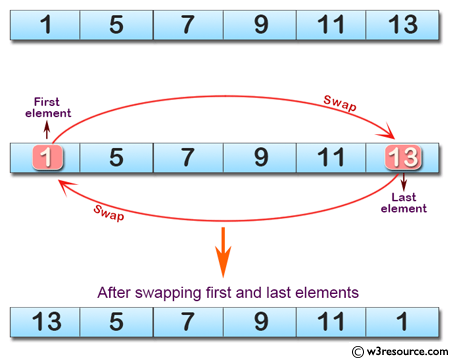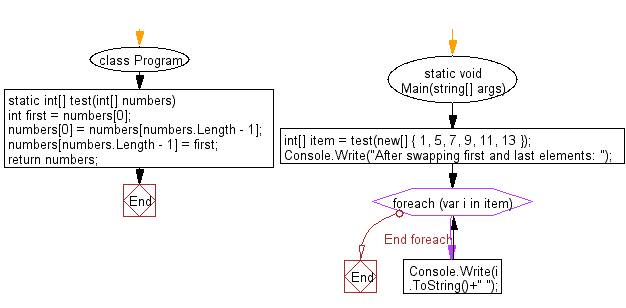﻿ C# - Swap the first and last elements of an array# C# Sharp Basic Algorithm Exercises: Create a new array swapping the first and last elements of a given array of integers and length will be least 1

## C# Sharp Basic Algorithm: Exercise-105 with Solution

Write a C# Sharp program to create a new array swapping the first and last elements of a given array of integers and length will be least 1.

Pictorial Presentation:Sample Solution:-

C# Sharp Code:

``````using System;
namespace exercises
{
class Program
{
static void Main(string[] args)
{
int[] item = test(new[] { 1, 5, 7, 9, 11, 13 });
Console.Write("After swapping first and last elements: ");
foreach(var i in item)
{
Console.Write(i.ToString()+" ");
}
}
static int[] test(int[] numbers)
{
int first = numbers;
numbers = numbers[numbers.Length - 1];
numbers[numbers.Length - 1] = first;

return numbers;
}
}
}
```
```

Sample Output:

`After swapping first and last elements: 13 5 7 9 11 1`

Flowchart:C# Sharp Code Editor:

Improve this sample solution and post your code through Disqus

What is the difficulty level of this exercise?

Test your Programming skills with w3resource's quiz.

﻿